# Search by Topic

Filter by: Content type:
Age range:
Challenge level:

### There are 67 results

Broad Topics > NC > NC Yr 5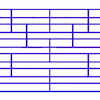### Tumbling Down

##### Age 7 to 11 Challenge Level:

Watch this animation. What do you see? Can you explain why this happens?### Pouring Problem

##### Age 7 to 11 Challenge Level:

What do you think is going to happen in this video clip? Are you surprised?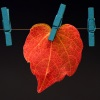### Number Lines in Disguise

##### Age 7 to 14 Challenge Level:

Some of the numbers have fallen off Becky's number line. Can you figure out what they were?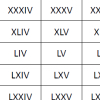### Roman Numerals

##### Age 7 to 11 Challenge Level:

Can you find some examples when the number of Roman numerals is fewer than the number of Arabic numerals for the same number?### Space Distances

##### Age 7 to 11 Challenge Level:

This task focuses on distances travelled by the asteroid Florence. It's an opportunity to work with very large numbers.### A4 Fraction Subtraction

##### Age 7 to 11 Challenge Level:

This task offers opportunities to subtract fractions using A4 paper.### A4 Fraction Addition

##### Age 7 to 11 Challenge Level:

Try adding fractions using A4 paper.### Subtraction Surprise

##### Age 7 to 14 Challenge Level:

Try out some calculations. Are you surprised by the results?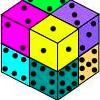### Six Numbered Cubes

##### Age 7 to 11 Challenge Level:

This task combines spatial awareness with addition and multiplication.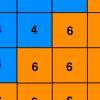### Six Ten Total

##### Age 7 to 11 Challenge Level:

This challenge combines addition, multiplication, perseverance and even proof.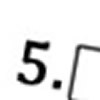### Greater Than or Less Than?

##### Age 7 to 11 Challenge Level:

Use the numbers and symbols to make this number sentence correct. How many different ways can you find?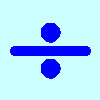### Division Rules

##### Age 7 to 11 Challenge Level:

This challenge encourages you to explore dividing a three-digit number by a single-digit number.### Multiply Multiples 3

##### Age 7 to 11 Challenge Level:

Have a go at balancing this equation. Can you find different ways of doing it?### Round the Dice Decimals 2

##### Age 7 to 11 Challenge Level:

What happens when you round these numbers to the nearest whole number?### Multiply Multiples 2

##### Age 7 to 11 Challenge Level:

Can you work out some different ways to balance this equation?### Multiply Multiples 1

##### Age 7 to 11 Challenge Level:

Can you complete this calculation by filling in the missing numbers? In how many different ways can you do it?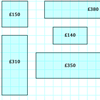### Through the Window

##### Age 7 to 11 Challenge Level:

My local DIY shop calculates the price of its windows according to the area of glass and the length of frame used. Can you work out how they arrived at these prices?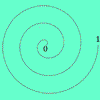### Spiralling Decimals

##### Age 7 to 14 Challenge Level:

Take turns to place a decimal number on the spiral. Can you get three consecutive numbers?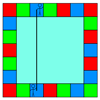### Ribbon Squares

##### Age 7 to 11 Challenge Level:

What is the largest 'ribbon square' you can make? And the smallest? How many different squares can you make altogether?### Olympic Turns

##### Age 7 to 11 Challenge Level:

This task looks at the different turns involved in different Olympic sports as a way of exploring the mathematics of turns and angles.### Factor Track

##### Age 7 to 14 Challenge Level:

Factor track is not a race but a game of skill. The idea is to go round the track in as few moves as possible, keeping to the rules.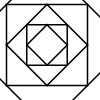### Shaping It

##### Age 5 to 11 Challenge Level:

These pictures were made by starting with a square, finding the half-way point on each side and joining those points up. You could investigate your own starting shape.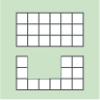### Area and Perimeter

##### Age 7 to 11 Challenge Level:

What can you say about these shapes? This problem challenges you to create shapes with different areas and perimeters.### Curious Number

##### Age 7 to 11 Challenge Level:

Can you order the digits from 1-3 to make a number which is divisible by 3 so when the last digit is removed it becomes a 2-figure number divisible by 2, and so on?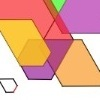### The Numbers Give the Design

##### Age 7 to 11 Challenge Level:

Make new patterns from simple turning instructions. You can have a go using pencil and paper or with a floor robot.### Three Dice

##### Age 7 to 11 Challenge Level:

Investigate the sum of the numbers on the top and bottom faces of a line of three dice. What do you notice?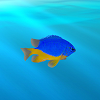### Sea Level

##### Age 7 to 11 Challenge Level:

The picture shows a lighthouse and many underwater creatures. If you know the markings on the lighthouse are 1m apart, can you work out the distances between some of the different creatures?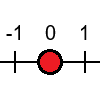### Tug Harder!

##### Age 7 to 11 Challenge Level:

In this game, you can add, subtract, multiply or divide the numbers on the dice. Which will you do so that you get to the end of the number line first?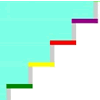### Swimming Pool

##### Age 5 to 11 Challenge Level:

In this problem, we're investigating the number of steps we would climb up or down to get out of or into the swimming pool. How could you number the steps below the water?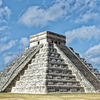### Picture a Pyramid ...

##### Age 7 to 11 Challenge Level:

Imagine a pyramid which is built in square layers of small cubes. If we number the cubes from the top, starting with 1, can you picture which cubes are directly below this first cube?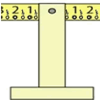### Balance of Halves

##### Age 7 to 11 Challenge Level:

Investigate this balance which is marked in halves. If you had a weight on the left-hand 7, where could you hang two weights on the right to make it balance?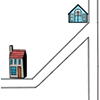### Six Places to Visit

##### Age 7 to 11 Challenge Level:

Can you describe the journey to each of the six places on these maps? How would you turn at each junction?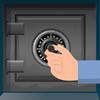### How Safe Are You?

##### Age 7 to 11 Challenge Level:

How much do you have to turn these dials by in order to unlock the safes?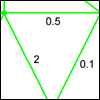### Route Product

##### Age 7 to 11 Challenge Level:

Find the product of the numbers on the routes from A to B. Which route has the smallest product? Which the largest?### Factors and Multiples Game

##### Age 7 to 16 Challenge Level:

A game in which players take it in turns to choose a number. Can you block your opponent?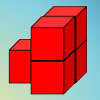##### Age 7 to 11 Challenge Level:

How can you arrange the 5 cubes so that you need the smallest number of Brush Loads of paint to cover them? Try with other numbers of cubes as well.### More Transformations on a Pegboard

##### Age 7 to 11 Challenge Level:

Use the interactivity to find all the different right-angled triangles you can make by just moving one corner of the starting triangle.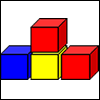### Up and Down Staircases

##### Age 7 to 11 Challenge Level:

One block is needed to make an up-and-down staircase, with one step up and one step down. How many blocks would be needed to build an up-and-down staircase with 5 steps up and 5 steps down?### Odd Squares

##### Age 7 to 11 Challenge Level:

Think of a number, square it and subtract your starting number. Is the number you’re left with odd or even? How do the images help to explain this?### Trebling

##### Age 7 to 11 Challenge Level:

Can you replace the letters with numbers? Is there only one solution in each case?### Fitted

##### Age 7 to 11 Challenge Level:

Nine squares with side lengths 1, 4, 7, 8, 9, 10, 14, 15, and 18 cm can be fitted together to form a rectangle. What are the dimensions of the rectangle?### Which Is Quicker?

##### Age 7 to 11 Challenge Level:

Which is quicker, counting up to 30 in ones or counting up to 300 in tens? Why?### Transformations on a Pegboard

##### Age 7 to 11 Challenge Level:

How would you move the bands on the pegboard to alter these shapes?### Matching Fractions, Decimals and Percentages

##### Age 7 to 14 Challenge Level:

An activity based on the game 'Pelmanism'. Set your own level of challenge and beat your own previous best score.### Cubes Within Cubes

##### Age 7 to 14 Challenge Level:

We start with one yellow cube and build around it to make a 3x3x3 cube with red cubes. Then we build around that red cube with blue cubes and so on. How many cubes of each colour have we used?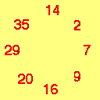### Cycling Squares

##### Age 7 to 11 Challenge Level:

Can you make a cycle of pairs that add to make a square number using all the numbers in the box below, once and once only?### Two Primes Make One Square

##### Age 7 to 11 Challenge Level:

Can you make square numbers by adding two prime numbers together?### Multiplication Squares

##### Age 7 to 11 Challenge Level:

Can you work out the arrangement of the digits in the square so that the given products are correct? The numbers 1 - 9 may be used once and once only.### Reach 100

##### Age 7 to 14 Challenge Level:

Choose four different digits from 1-9 and put one in each box so that the resulting four two-digit numbers add to a total of 100.### All the Digits

##### Age 7 to 11 Challenge Level:

This multiplication uses each of the digits 0 - 9 once and once only. Using the information given, can you replace the stars in the calculation with figures?